Back to homepage

# Introduction

Gnuplot is an open source program used to plot two or three dimensional graphs.
```It can operate in interactive mode, or read commands and data from files.
Here are some basic use examples to get you started.

Type in:
gnuplot

to start the program.
You will be presented with intro screen and interactive command prompt (gnuplot>).
You can type help or this formula to get you started:

gnuplot> plot sin(x)

Another graphical window will be created, with sin(x) plotted.
You can copy that to clipboard etc.

Now try:
gnuplot> plot f(x)=x
^
function to plot expected

We get error message. For some odd reason, this works:

gnuplot> plot f(x)=x,f(x)

and makes a nice graph in a separate window.
```

# Multiple graphs

Now let's make a nicer, more complicated graph:
```gnuplot> plot f(x)=(x*x)/(1-x),f(x)
```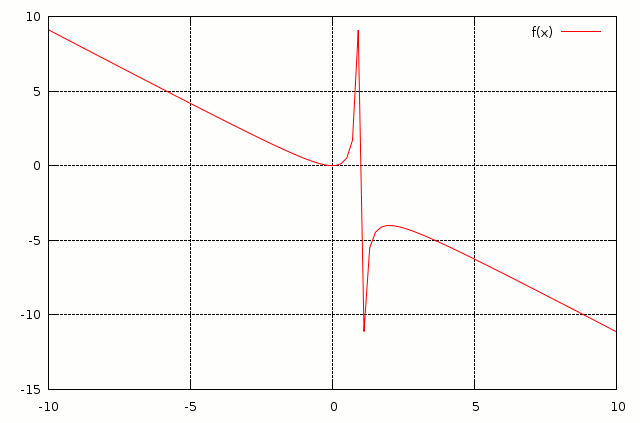Graph of function f(x)=(x*x)/(1-x).

```There can be several functions plotted on the same graph.
Here we plot sin(x) functions with three different periods: one, two and four:

gnuplot> plot f(x)=sin(x*b),b=1,f(x),b=0.5,f(x),b=0.25,f(x)
```Three sin(x) functions with different periods drawn on the same graph.

```Harmonic addition of those three individual functions:
gnuplot> plot f(x)=sin(x*b)+sin(x*0.5*b)+sin(x*0.25*b),b=1,f(x)
```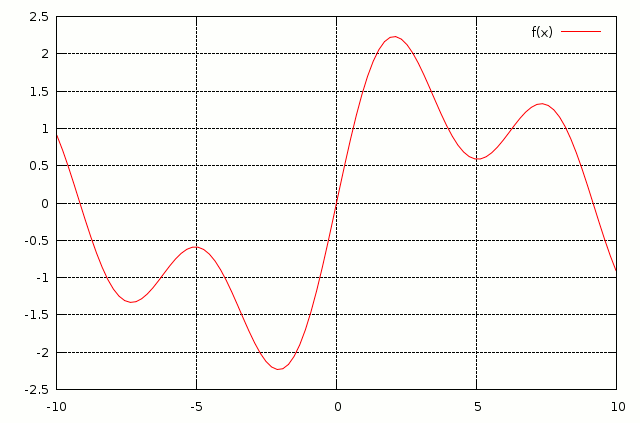Harmonic addition of three functions.

# Range of display and tick increments

```To ensure that only certain horizontal range is shown, enter range before function:
gnuplot> plot [-20:20] sin(x)+cos(x)+tan(x)
```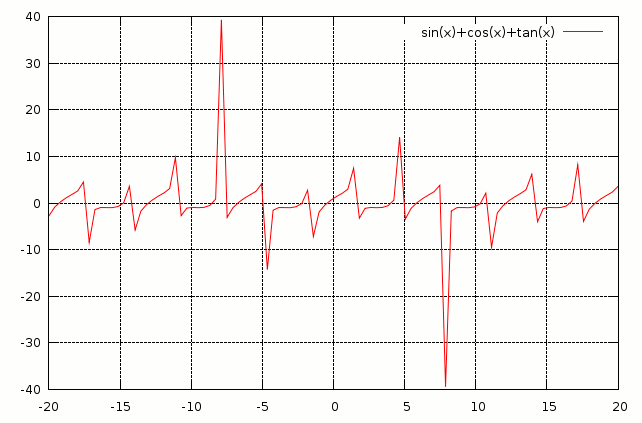Only given horizontal range (-20:20) of function is plotted.

```To set particular number increments for x and y axis:
gnuplot> set xtics pi
gnuplot> set ytics 5

Then:
gnuplot> plot [-2*pi:2*pi] (sin(x)+cos(x))*tan(x)
```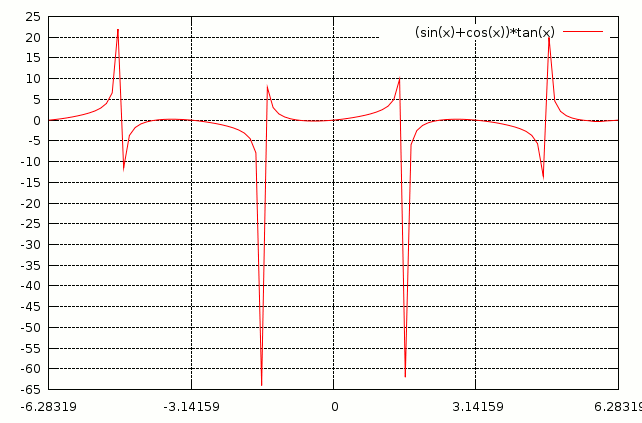How to specify particular increments for x and y axis.

# 3D (three dimensional) plots

```Now for 3D (three-dimensional) plot with set x and y ranges and tics:
gnuplot> set xtics 0.5

gnuplot> set ytics 0.5
gnuplot> splot [x=-5:5] [y=-5:5] sin(x) * cos(y)
```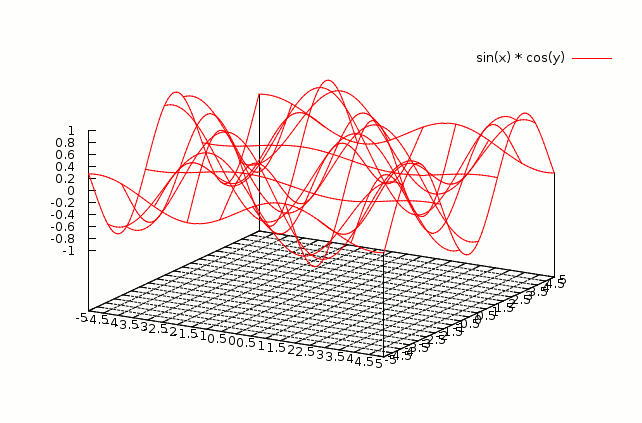Basic 3D plot.

```Filled up color plot:
gnuplot> set xlabel "X"
gnuplot> set ylabel "Y"
gnuplot> set zlabel "Z"
gnuplot> set xtics 0.5
gnuplot> set ytics 0.5
gnuplot> set ztics 0.5
gnuplot> set pm3d
gnuplot> set hidden3d
gnuplot> splot [x=-3:3] [y=-3:3] sin(x) * (0.3*tan(y))
```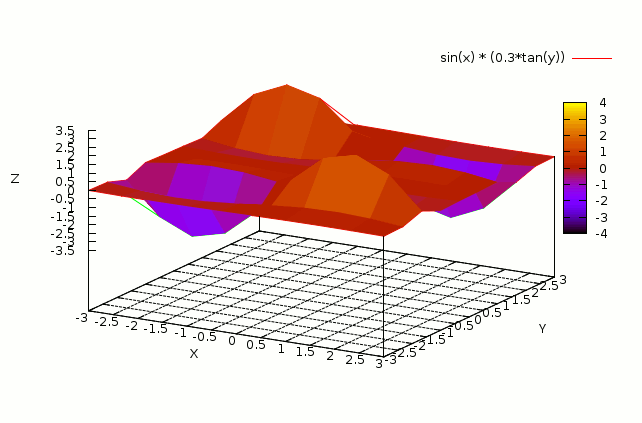This gives 3D colour plot.

# Plotting data from text files

```Plotting data from files using Gnuplot:
gnuplot> plot "testdata1.tsv" using 1:2
```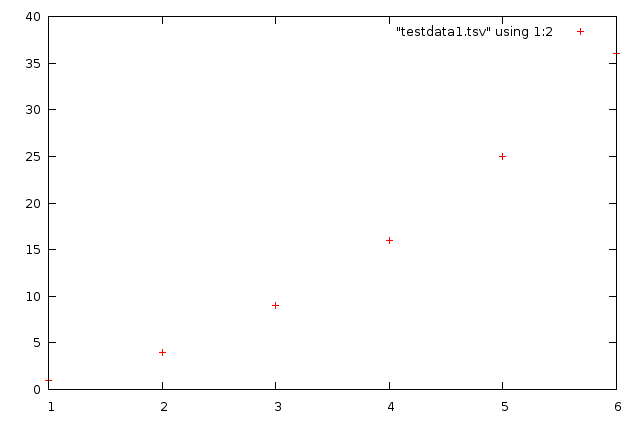This will only work if you have already prepared a human readable text file with values.
Here is one to get you started.
It is using tab delimeters for data, and line with # at the beginning is ignored.

# Useful links

Collection of excellent examples at http://gnuplot.sourceforge.net/demo/Back to homepage